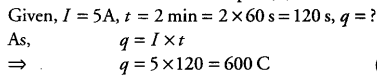# What is meant by electric current? Write its SI unit. Calculate the amount of charge that flows

What is meant by electric current? Write its SI unit. Calculate the amount of charge that flows through a conductor when a current of 5 amperes flows through it for 2 minutes.

Electric current is defined as the rate of flow of electric charge through any cross-section of a conductor.
SI unit of electric current is ampere(A).Thus, the amount of charge flowing through conductor is 600 C.